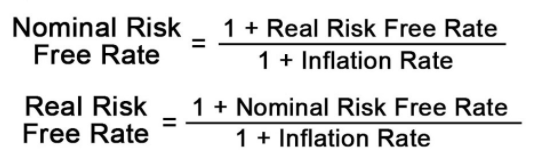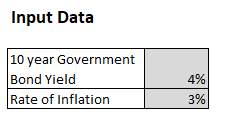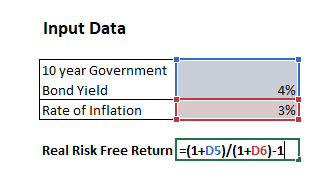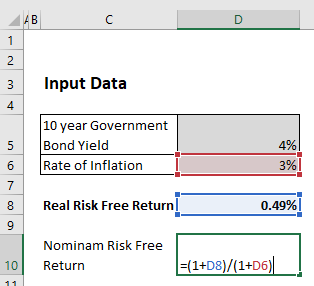#### How to calculate risk-free rate in Excel

A risk-free rate is the rate of interest a borrower has to pay or an investor expects to earn on an asset carrying zero risks. It is the minimum return that an investment must give over a period to be profitable. If you keep the money in a fixed deposit, you will get an assured interest. A risk free rate is like that and can be calculated by using a mathematical formula.

Generally, the central bank of a country guarantees a risk-free rate on government bonds or bank deposits.

It is assumed that bonds of developed countries do not have a default risk. There is a currency risk when investing in bonds or deposits in a different currency. For this reason, investments in bonds or foreign currency deposits cannot be considered as investments with a zero risk rate.

## Real vs Nominal Risk-Free Rate

The nominal risk-free interest rate does not consider inflation. It is the stated interest on a loan or return on an investment without considering macroeconomic impacts or compounding of interest.

The real risk-free rate is adjusted for the effects of inflation. It reflects the real cost that the borrower withdraws when the investor invests.

The real risk-free rate should be considered while making a business decision for the profitability of a project.

## Conversion between Real and Nominal Risk-Free Rate## Risk-Free Interest Rate

Risk Free Rate of Return Formula = (1+ Government Bond Rate)/ (1+Inflation Rate)-1

## Calculate Using Excel

Step 1: Insert the input data available from the website of the central bank of your country.Step 2: Calculate the Real Rate using the formula.Risk Free Rate of Return Formula = (1+ Government Bond Rate)/ (1+Inflation Rate)-1

Step 3: Calculate Nominal Risk-Free Rate.

The formula is: Nominal Risk Free Rate of Return Formula = (1+ Real Risk free rate)/ (1+Inflation Rate)The risk-free rate is used to calculate the Capital Asset Pricing Model and the Weighted Average Cost of Capital.

## Alternative method using RRi function

Another method to calculate the risk-free rate in Excel is to use the RRI function. The RRI function calculates the interest rate for an investment that grows by a specified amount over a specified number of periods. To use this function, you will need to know the present value of the investment, the future value of the investment, and the number of periods.

Here are the steps to calculate the risk-free rate using the RRI function:

1. Determine the present value and future value: The present value is the initial investment amount, and the future value is the amount that the investment will be worth after a certain number of periods.
2. Determine the number of periods: The number of periods is the length of time that the investment will be held, expressed in the same unit as the interest rate (e.g., months, years).
3. Enter the data into Excel: In a new spreadsheet, enter the present value in one cell, the future value in another cell, and the number of periods in a third cell.
4. Use the RRI function: In a fourth cell, use the RRI function to calculate the interest rate. The formula is: =RRI(number of periods, future value/present value).
5. Adjust for the frequency of compounding: If the interest rate is not quoted on an annual basis, you will need to adjust it to an annual rate. For example, if the interest rate is quoted on a semi-annual basis, you would multiply it by 2 to get the annual rate.
6. Use the risk-free rate in your financial models: You can use the calculated risk-free rate as a benchmark for evaluating the risk of other investments in various financial models.

By following these steps, you can use the RRI function in Excel to calculate the risk-free rate and use it as a benchmark for evaluating the risk of other investments. Note that the risk-free rate is subject to change over time, so it is important to regularly update your calculations to reflect the most current information.

## Alternative method using the bond yield formula

Another method to calculate the risk-free rate in Excel is to use the bond yield formula. The bond yield formula calculates the return on a bond based on its current market price and coupon rate. To use this method, you will need to know the bond’s coupon rate, its face value, and its market price.

Here are the steps to calculate the risk-free rate using the bond yield formula:

1. Determine the bond’s coupon rate: The coupon rate is the annual interest payment that the bond pays. It is expressed as a percentage of the bond’s face value.
2. Determine the bond’s face value: The face value of a bond is the amount that the bond will be worth when it matures.
3. Determine the bond’s market price: The market price of a bond is the current price that the bond is trading for in the market.
4. Enter the data into Excel: In a new spreadsheet, enter the coupon rate in one cell, the face value in another cell, and the market price in a third cell.
5. Use the bond yield formula: In a fourth cell, use the bond yield formula to calculate the interest rate. The formula is: =(Coupon Rate / 2) / (1 – (Market Price / Face Value)) + (Coupon Rate / 2).
6. Adjust for the frequency of compounding: If the interest rate is not quoted on an annual basis, you will need to adjust it to an annual rate. For example, if the interest rate is quoted on a semi-annual basis, you would multiply it by 2 to get the annual rate.
7. Use the risk-free rate in your financial models: You can use the calculated risk-free rate as a benchmark for evaluating the risk of other investments in various financial models.